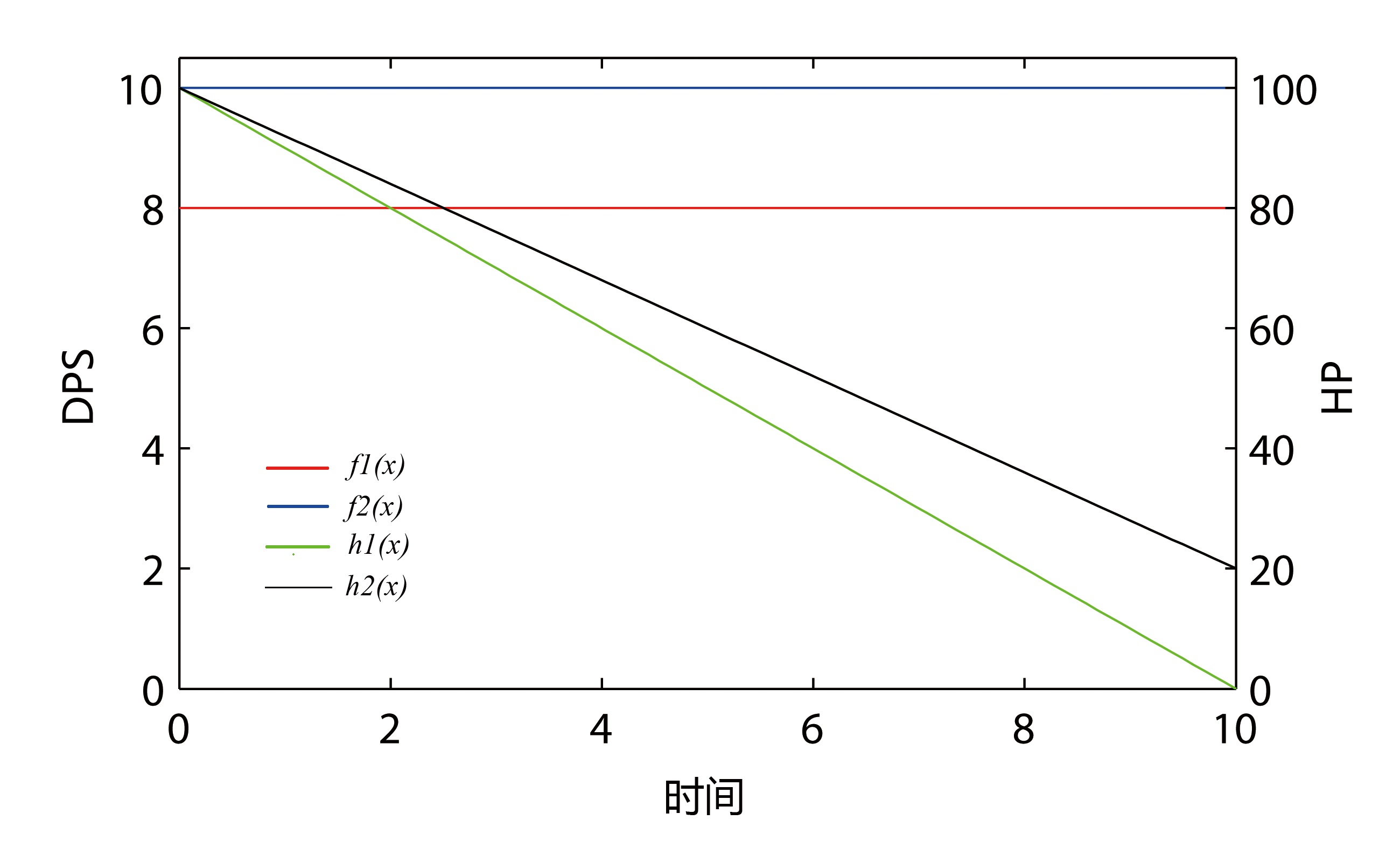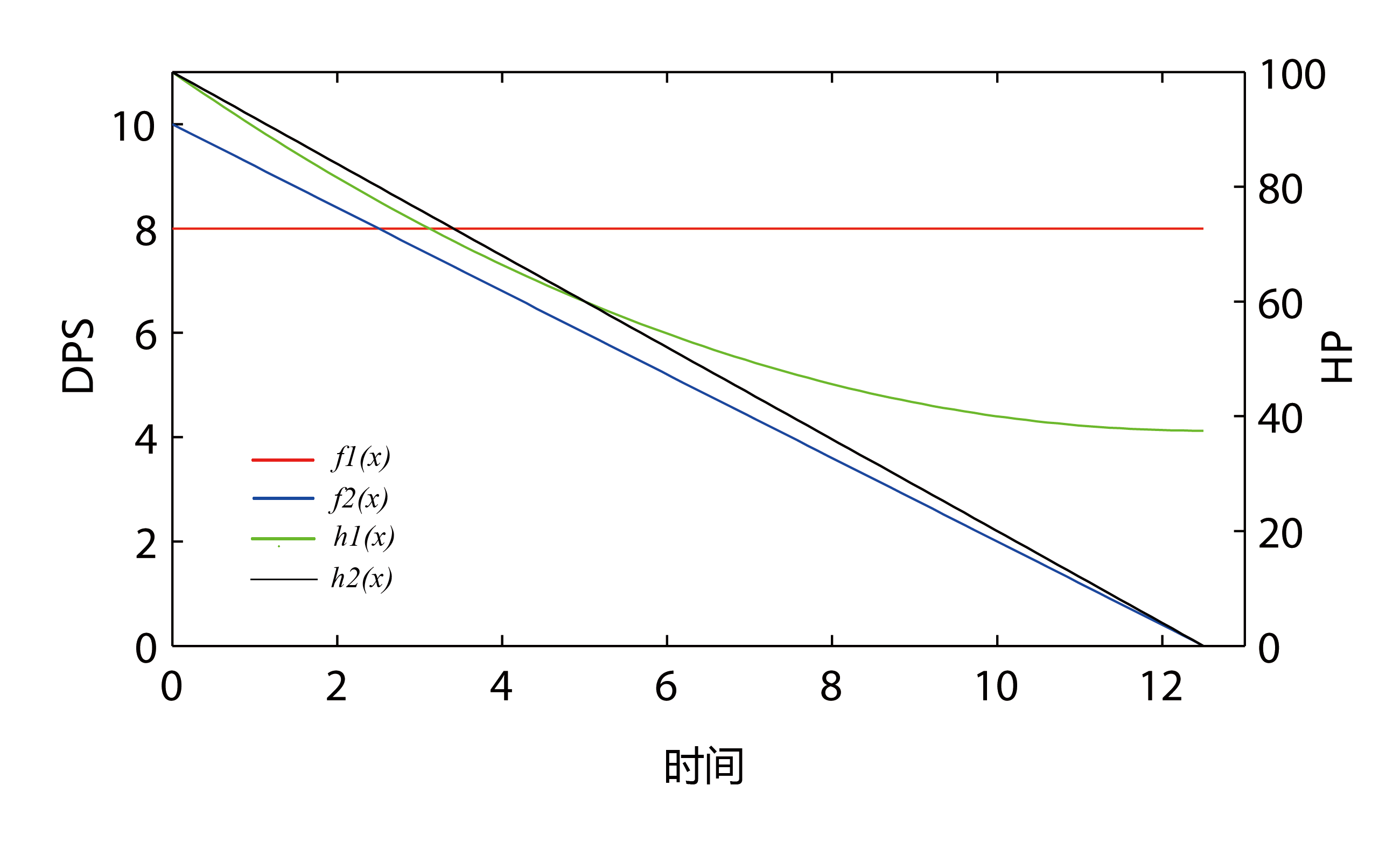# 游戏中的数学模型——战斗过程篇

## 0x00 符号声明

A 某个战斗单位通过一系列的基于游戏机制的运算，得到这个战斗单位的基本DPS （Damage Per Second）。对于由若干单位组成的部队，其值是各个单位DPS之和。

H 某个战斗单位的总生命值（能够承受伤害的总值）。对于由若干单位组成的部队，其值是各个单位生命值之和。

f(t) f(t) 在本文中表示某个战斗单位的实际DPS随战斗时间变化的函数。

h(t) h(t) 在本文中表示某个战斗单位的实际生命值随战斗时间变化的函数。

$$h(t) = H - \Delta H = H - \int_ {0}^{t} f(x)dx$$

（式1) 注意：此处f(x)为敌方的f(t)

## 0x01 一个简单的情况

$$f_1(t) = A_1$$
$$f_2(t) = A_2$$

$$h_1(t) = H_1 - A_2t$$
$$h_2(t) = H_2 - A_1t$$## 0x02 一个有点复杂的情况

$$f(t) = \frac {h(t)} {H} A$$

(式 2)

$$f_1(t) = A_1$$
$$f_2(t) = \frac {h_2(t)} {H_2} A_2 = A_2 \frac{H_2 - \int_ {0}^{t} f_1(x)dx}{H_2} = A_2(1 - \frac{A_1t}{H_2})$$

$$h_1(t) = H_1 - A_2(t - \frac{A_1}{2H_2} t^2)$$
$$h_2(t) = H_2 - A_1t$$## 0x03 一个棘手的情况

$$f_1 (t)=A_1 \frac {H_1 - \int_{0}^{t} f_2(x) dx} {H_1}$$
$$f_2 (t)=A_2 \frac {H_2 - \int_{0}^{t} f_1(x) dx} {H_2}$$

$$f_1(t)=A_1- \frac {A_1}{H_1} \int_ {0}^{t}(A_2-\frac{A_2}{H_2} \int_ {0}^{x} f_1(y)dy)dx$$

$$f_1(t)=A_1cosh(\sqrt{\frac{A_1A_2}{H_1H_2}}t)-A_2\sqrt{\frac{H_2A_1}{H_1A_2}}sinh(\sqrt{\frac{A_1A_2}{H_1H_2}}t)$$
$$f_2(t)=A_2cosh(\sqrt{\frac{A_1A_2}{H_1H_2}}t)-A_1\sqrt{\frac{H_1A_2}{H_2A_1}}sinh(\sqrt{\frac{A_1A_2}{H_1H_2}}t)$$

$$\lambda = \sqrt{\frac{A_1A_2}{H_1H_2}}$$

$$f_1(t)=A_1cosh(\lambda t)-\lambda H_2sinh(\lambda t)$$

$$f_2(t)=A_2cosh(\lambda t)-\lambda H_1 sinh(\lambda t)$$

$$h_1(t)= H_1cosh(\lambda t) - \frac{A_2}{\lambda} sinh(\lambda t)$$
$$h_2(t)= H_2cosh(\lambda t) - \frac{A_1}{\lambda} sinh(\lambda t)$$